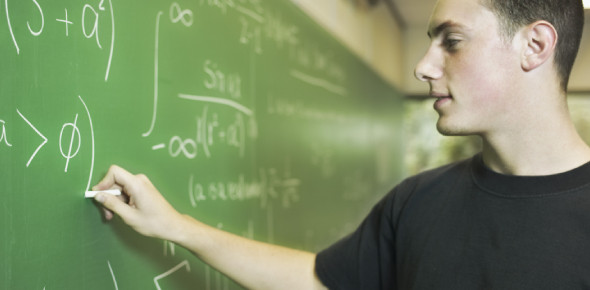# College Math Quiz Questions And Answers

10 Questions | Total Attempts: 11550SettingsHere is an amazing math quiz for you. Get ready for this College math quiz. Do you think you can pass the college math exam? One of the most imperative subjects you will ever study in school s math. For this quiz, you are likely to know algebra 2, geometry, or pre-calculus. This quiz will show you that college math isn’t a scary subject. You can do it. Go for it.

• 1.
Find the value of 2[3(x^2 - 5) + 5y] when x = 9 and y = 3.
• A.

729

• B.

486

• C.

429

• D.

629

• 2.
If the sum of the first two of the five consecutive odd integers is 52, what is the sum of the last two integers?
• A.

61

• B.

67

• C.

64

• D.

58

• 3.
Freight charges of a certain courier company are as follows: \$40 for the first 2 pounds \$15 for each of the succeeding pound weight of parcels. At this rate, what amount would be charged on a package which weighs 30 pounds?
• A.

\$450

• B.

\$460

• C.

\$640

• D.

\$600

• 4.
If 5/6 < x and x < 7/4 then x could be ____.
• A.

1/2

• B.

3/2

• C.

2/3

• D.

2

• 5.
If a square's each side is increased by x units, then, after that, its perimeter is increased by how many units?
• A.

2x

• B.

X

• C.

8x

• D.

4x

• 6.
One day, Mr. Paul left the office at 8:35 PM and drove to his home. If he reached there at 10:02 PM, how long did he travel?
• A.

1 hour and 33 minutes.

• B.

1 hour and 17 minutes

• C.

1 hour and 27 minutes.

• D.

2 hours and 33 minutes

• 7.
Which of the following is more than 0.35 but less than 0.41?
• A.

8/25

• B.

3/10

• C.

2/5

• D.

9/26

• 8.
Which of the following is not greater than 7/16?
• A.

1/2

• B.

31/62

• C.

15/17

• D.

3/8

• 9.
When simplified, |9+(4-3)-17| has a value of _____.
• A.

-7

• B.

13

• C.

9

• D.

7

• 10.
When a = 4 and b = 3/7, which of the following expressions represents the smallest value?
• A.

59/7

• B.

22/7

• C.

28/3

• D.

3/28

Related TopicsBack to top